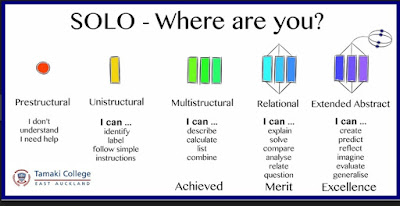## Sunday, June 23, 2019

### GO SOLO Yr13

Systems of Equations As91587(3.15)Using SOLO taxonomy involves learners in their own differentiation and makes the
process behind learning explicit. It highlights the difference between surface and deep
understanding, helping students understand where they are on that spectrum, and what
they need to do to progress.
My learners found SOLO taxonomy very useful to understand the NCEA breakdown A/M/E

 Achievement Form the 3 simultaneous equations Find a solution to the simultaneous equation Interpret the valid solution in context Merit Defined the variables Justified the valid discrete solution Formed new equations Shown algebraically solved 0=0 and interpreted the  solutions Found the relationship between variables and filling equations and interpreted Excellence Shown algebraically solved 0=0 and interpreted the  solutions Found the relationship between variables and filling equations and interpreted Find the equation representing line of solutions Find a combination of values  for the variables that satisfies the model

Next step: Working on accuracy, Algebraic skill (3x3 Equations)